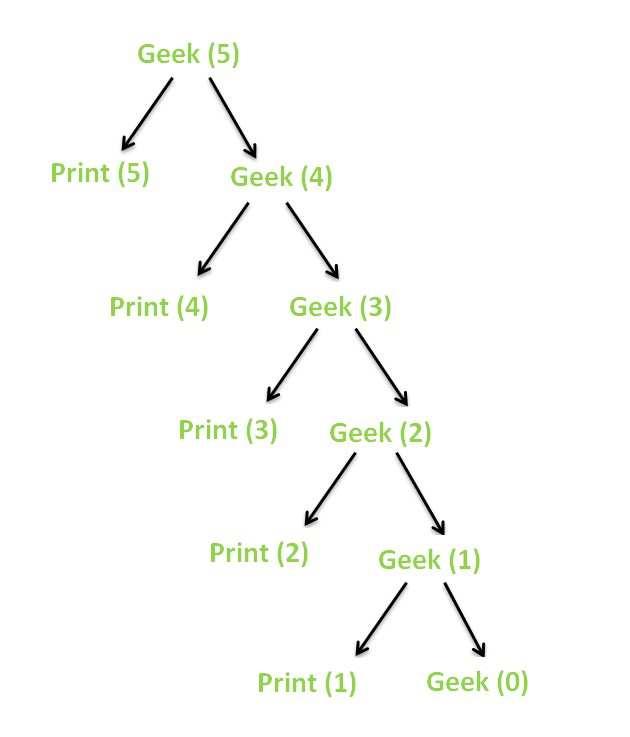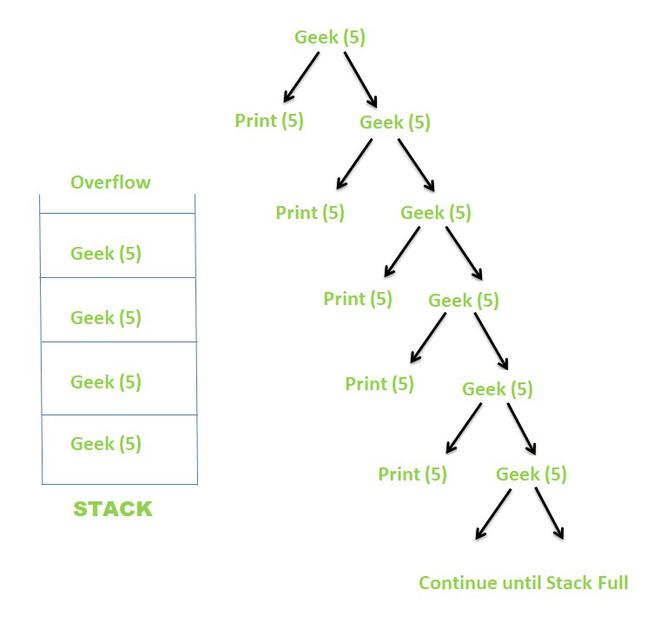GFG App
Open AppBrowser
Continue

# Finite and Infinite Recursion with examples

The process in which a function calls itself directly or indirectly is called Recursion and the corresponding function is called a Recursive function
Using Recursion, certain problems can be solved quite easily. Examples of such problems are Towers of Hanoi (TOH), Inorder/Preorder/Postorder Tree Traversals, DFS, etc.

Types of Recursions:

Recursion can be further classified into two kinds, depending on when they terminate:

1. Finite Recursion
2. Infinite Recursion

Finite Recursion:

Finite Recursion occurs when the recursion terminates after a finite number of recursive calls. A recursion terminates only when a base condition is met.

Example:

Below is an implementation to demonstrate Finite Recursion.

## C++

 `// C++ program to demsonstrate Finite Recursion` `#include ` `using` `namespace` `std;`   `// Recursive function` `void` `Geek(``int` `N)` `{` `    ``// Base condition` `    ``// When this condition is met,` `    ``// the recursion terminates` `    ``if` `(N == 0)` `        ``return``;`   `    ``// Print the current value of N` `    ``cout << N << ``" "``;`   `    ``// Call itself recursively` `    ``Geek(N - 1);` `}`   `// Driver code` `int` `main()` `{`   `    ``// Initial value of N` `    ``int` `N = 5;`   `    ``// Call the recursive function` `    ``Geek(N);` `    ``return` `0;` `}`

## Java

 `// Java program for the above approach` `class` `GFG{`   `// Recursive function` `static` `void` `Geek(``int` `N)` `{` `    `  `    ``// Base condition` `    ``// When this condition is met,` `    ``// the recursion terminates` `    ``if` `(N == ``0``)` `        ``return``;`   `    ``// Print the current value of N` `    ``System.out.println(N + ``" "``);`   `    ``// Call itself recursively` `    ``Geek(N - ``1``);` `}`   `// Driver code` `public` `static` `void` `main(String[] args)` `{` `    `  `    ``// Initial value of N` `    ``int` `N = ``5``;`   `    ``// Call the recursive function` `    ``Geek(N);` `}` `}`   `// This code is contributed by abhinavjain194`

## Python3

 `# Python program to demsonstrate Finite Recursion` `# Recursive function` `def` `Geek( N):`   `    ``# Base condition` `    ``# When this condition is met,` `    ``# the recursion terminates` `    ``if` `(N ``=``=` `0``):` `        ``return`   `    ``# Pr the current value of N` `    ``print``( N, end ``=``" "` `)`   `    ``# Call itself recursively` `    ``Geek(N ``-` `1``)`     `# Driver code` `# Initial value of N` `N ``=` `5`   `# Call the recursive function` `Geek(N)`   `# this code is contributed by shivanisinghss2110`

## C#

 `// C# program for the above approach` `using` `System;` `using` `System.Collections.Generic;`   `class` `GFG{`   `// Recursive function` `static` `void` `Geek(``int` `N)` `{` `     `  `    ``// Base condition` `    ``// When this condition is met,` `    ``// the recursion terminates` `    ``if` `(N == 0)` `        ``return``;` ` `  `    ``// Print the current value of N` `    ``Console.Write(N + ``" "``);` ` `  `    ``// Call itself recursively` `    ``Geek(N - 1);` `}`   `// Driver Code` `public` `static` `void` `Main(String[] args)` `{` `  `  `    ``// Initial value of N` `    ``int` `N = 5;` ` `  `    ``// Call the recursive function` `    ``Geek(N);` `}` `}`   `// This code is contributed by target_2.`

## Javascript

 ``

Output

`5 4 3 2 1 `

Time Complexity: O(n)
Auxiliary Space: O(n)

The recursion tree for the above recursive function looks like this.Recursion Tree

When the value of N becomes 0, because of the base condition, the recursion terminates.

Infinite Recursion:

Infinite Recursion occurs when the recursion does not terminate after a finite number of recursive calls. As the base condition is never met, the recursion carries on infinitely.

Example:

Below is an implementation to demonstrate Infinite Recursion.

## C++

 `// C++ program to demsonstrate Infinite Recursion` `#include ` `using` `namespace` `std;`   `// Recursive function` `void` `Geek(``int` `N)` `{` `    ``// Base condition` `    ``// This condition is never met here` `    ``if` `(N == 0)` `        ``return``;`   `    ``// Print the current value of N` `    ``cout << N << ``" "``;`   `    ``// Call itself recursively` `    ``Geek(N);` `}`   `// Driver code` `int` `main()` `{`   `    ``// Initial value of N` `    ``int` `N = 5;`   `    ``// Call the recursive function` `    ``Geek(N);` `    ``return` `0;` `}`

## Java

 `// Java program to demsonstrate Infinite Recursion` `import` `java.io.*;` ` `  `class` `GFG` `{` `// Recursive function` `static` `void` `Geek(``int` `N)` `{` `    ``// Base condition` `    ``// This condition is never met here` `    ``if` `(N == ``0``)` `        ``return``;`   `    ``// Print the current value of N` `    ``System.out.print( N +``" "``);`   `    ``// Call itself recursively` `    ``Geek(N);` `}`   `// Driver code` `public` `static` `void` `main(String[] args)` `    ``{`   `    ``// Initial value of N` `    ``int` `N = ``5``;`   `    ``// Call the recursive function` `    ``Geek(N);` `    ``}` `}`   `// This code is contributed by shivanisinghss2110`

## Python3

 `# Python3 to demsonstrate Infinite Recursion`   `# Recursive function` `def` `Geek(N):` `    `  `    ``# Base condition` `    ``# This condition is never met here` `    ``if` `(N ``=``=` `0``):` `        ``return`   `    ``# Print the current value of N` `    ``print``(N, end ``=` `" "` `)`   `    ``# Call itself recursively` `    ``Geek(N)`   `# Driver code`   `# Initial value of N` `N ``=` `5`   `# Call the recursive function` `Geek(N)`   `# This code is contributed by shivanisinghss2110`

## C#

 `// C# program to demsonstrate Infinite Recursion` `using` `System;` ` `  `class` `GFG` `{` `// Recursive function` `static` `void` `Geek(``int` `N)` `{` `    ``// Base condition` `    ``// This condition is never met here` `    ``if` `(N == 0)` `        ``return``;`   `    ``// Print the current value of N` `    ``Console.Write( N +``" "``);`   `    ``// Call itself recursively` `    ``Geek(N);` `}`   `// Driver code` `public` `static` `void` `Main(String[] args)` `    ``{`   `    ``// Initial value of N` `    ``int` `N = 5;`   `    ``// Call the recursive function` `    ``Geek(N);` `    ``}` `}`   `// This code is contributed by shivanisinghss2110`

## Javascript

 ``

Time Complexity: non finite as this recursion will never end.
Auxiliary Space: non finite

The recursion tree for the above recursive function looks like this.Recursion Tree

Since the value of N never becomes 0, so the recursion never terminates. Instead, the recursion continues until the implicit stack becomes full which results in a Stack Overflow. Some compilers directly give the output as Segmentation Fault (Core Dumped), while others may abnormally terminate for some value and then show Segmentation fault

My Personal Notes arrow_drop_up